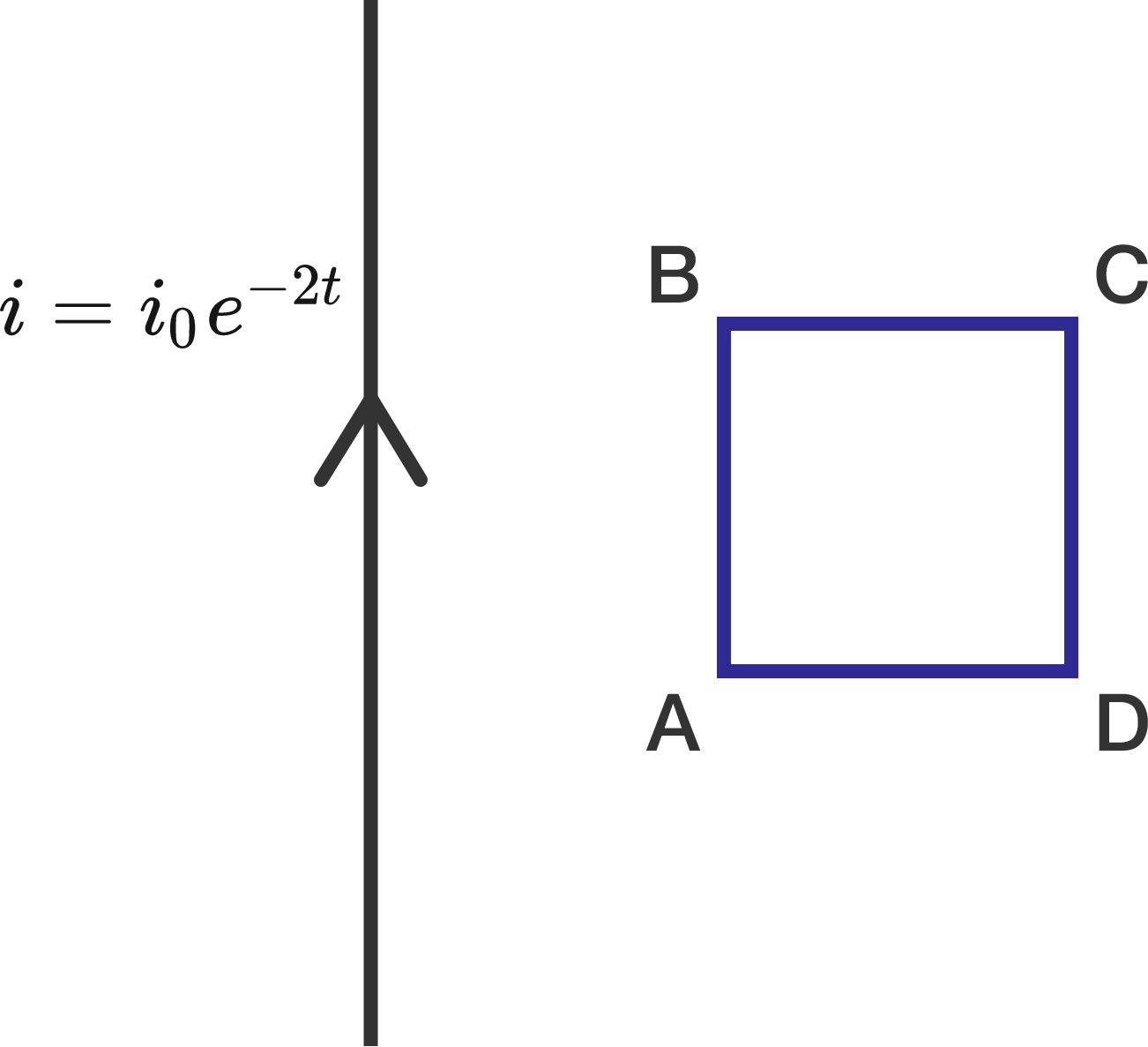What will this loop oppose?

A loop $ABCD$ is placed in the plane of an infinite current-carrying wire, as shown in the diagram.The current in the wire varies as $i=i_0 e^{-2t}$. What will be the direction of induced current in the loop?

×